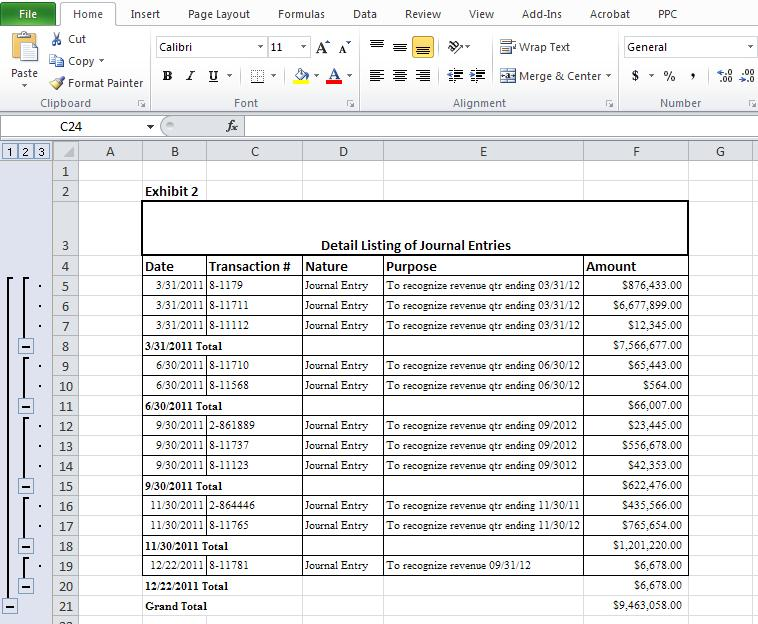# Subtotals Using Excel Subtotal Function

You can have Excel calculate subtotals for a portion of your worksheet data. Let me explain this with an exercise. With this exercise, let us assume we need to subtotal value of the journal entries processed each day of the month for the data on Exhibit 1 below:

Steps:

1. Click Data tab
2. Select the entire table
3. Click Subtotals
4. The sub total dialogue opens
5. Since we need to subtotal the amount of the journal entries processed on a particular day by date we go to the drop down arrow and select ‘at each change in’ ” Date”
6. For use function box we select “sum ” since we need to subtotal amounts
7. For add subtotal to box we select  ” amount” since  we need to arrive at total value at each change in date
8. Then select “Summary below date”
9. Click “OK”
10. See results in exhibit 2 belowFor further reading you may consider buying Microsoft® Office Excel® 2007: Data Analysis and Business Modeling book at any book store such as http://www.amazon.com depending on the version of Microsoft excel version you are using

This entry was posted in Microsoft Excel Simplified. Bookmark the permalink.